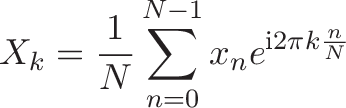# Fourier Transform

## Colorized Definition\newcommand{\energy}{\color{c1}}
\newcommand{\freq}{\color{c2}}
\newcommand{\spin}{\color{c3}}
\newcommand{\signal}{\color{c4}}
\newcommand{\Circle}{\color{c5}}
\newcommand{\average}{\color{c6}}$\displaystyle{\energy X_{\freq k} \plain = \average \frac{1}{N} \sum_{n=0}^{N-1} \signal x_n \spin e^{\mathrm{i} \Circle 2\pi \freq k \average \frac{n}{N}}}$

\plain     To find
\energy    the energy
\freq      at a particular frequency,
\spin      spin
\
\Circle    around a circle
\freq      at that frequency,
\plain     and
\
\average   average a bunch of points along that path.


## Plain English

• What does the Fourier Transform do? Given a smoothie, it finds the recipe.

• How? Run the smoothie through filters to extract each ingredient.

• Why? Recipes are easier to analyze, compare, and modify than the smoothie itself.

• How do we get the smoothie back? Blend the ingredients.

## Read More - [Interactive Guide to the Fourier Transform](https://betterexplained.com/articles/an-interactive-guide-to-the-fourier-transform/)